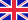•# The analysis of the time-dependent behavior of queues

This book studies the time-dependent behavior of queuing systems which has non-empty initial work load. The technique used to analyze the behavior of the queueing systems studied in this book is based on the Wiener-Hopf factorization. The first major step... Viac o knihe

Na objednávku, dodanie 2-4 týždne

71.54 €

bežná cena: 81.30 €

## O knihe

This book studies the time-dependent behavior of queuing systems which has non-empty initial work load. The technique used to analyze the behavior of the queueing systems studied in this book is based on the Wiener-Hopf factorization. The first major step in the analysis is the derivation of the (system of) transformed Wiener-Hopf equation(s). Wiener-Hopf factorization is then applied to its symbol. Since the queueing systems we consider have a non-zero initial working load, the Wiener-Hopf factorization should be followed by a decomposition on a certain (matrix) function. The Wiener-Hopf factorization and the decomposition yields a (formal) solution of the (system of) equation(s).If the stability condition is fulfilled, then the steady-state distributions of interest can be determined by applying Abel's limit theorem to the solution of the (system of) equation(s).In this book it is shown how the time-dependent distributions converge to the steady-state distribution.

• Vydavateľstvo: LAP LAMBERT Academic Publishing
• Rok vydania: 2011
• Formát: Paperback
• Rozmer: 220 x 150 mm
• Jazyk: Anglický jazyk
• ISBN: 9783844333275

Generuje redakčný systém BUXUS CMS spoločnosti ui42.